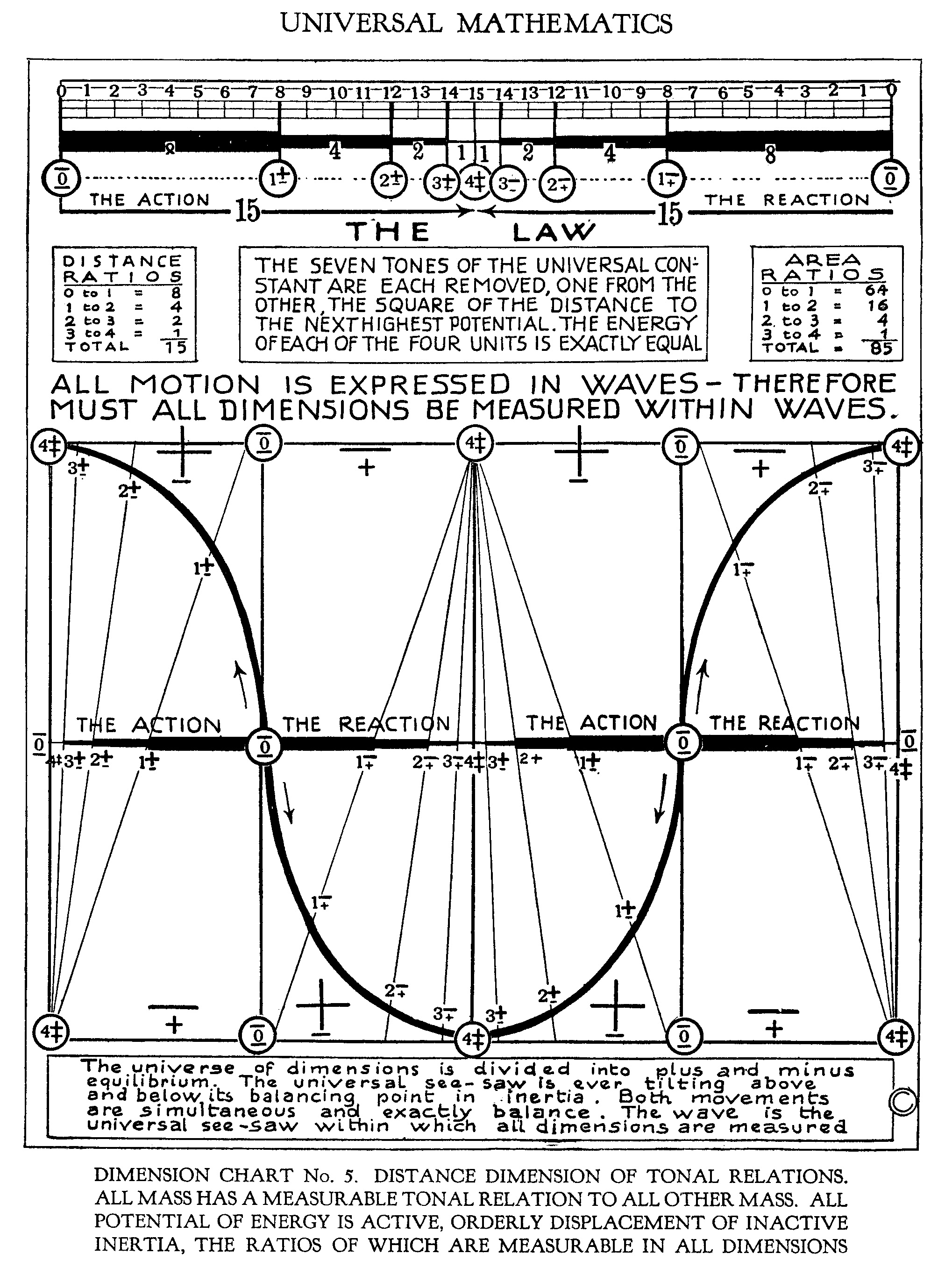# Dimension Chart 5THE SEVEN TONES OF THE UNIVERSAL CONSTANT ARE EACH REMOVED, ONE FROM THE OTHER, THE SQUARE OF THE DISTANCE TO THE NEXT HIGHEST POTENTIAL. THE ENERGY OF EACH OF THE FOUR UNITS IS EXACTLY EQUAL. [Dimension Chart 5]

ALL MOTION IS EXPRESSED IN WAVES - THEREFORE MUST ALL DIMENSIONS BE MEASURED WITHIN WAVES. [Dimension Chart 5]

The universe of dimensions is divided into plus and minus equilibrium. The universal seesaw is ever tilting above and below its balancing point in inertia. Both movements are simultaneous and exactly balance[d]. The wave is the universal seesaw within which all dimensions are measured. [Dimension Chart 5]

DIMENSION CHART No. 5. DISTANCE DIMENSION OF TONAL RELATIONS.
ALL MASS HAS A MEASURABLE TONAL RELATION TO ALL OTHER MASS. ALL POTENTIAL OF ENERGY IS ACTIVE. ORDERLY DISPLACEMENT OF INACTIVE INERTIA, THE RATIOS OF WHICH ARE MEASURABLE IN ALL DIMENSIONS [Dimension Chart 5]

Created by Dale Pond. Last Modification: Monday May 15, 2023 05:12:11 MDT by Dale Pond.